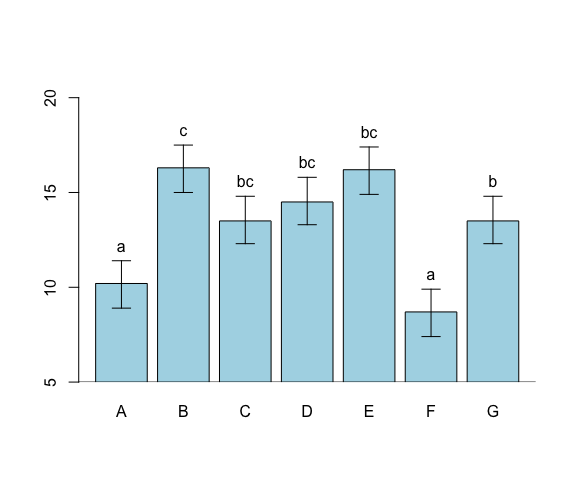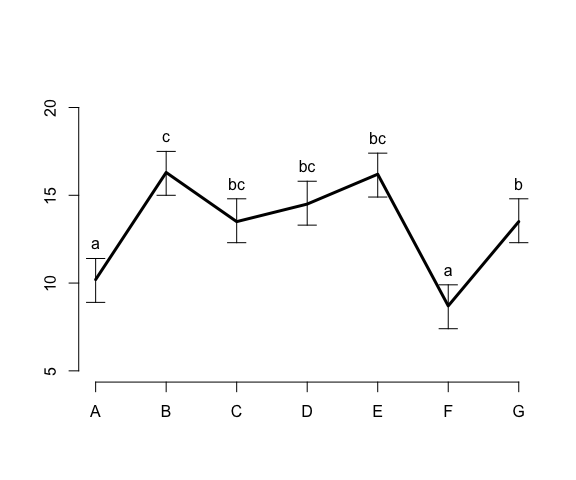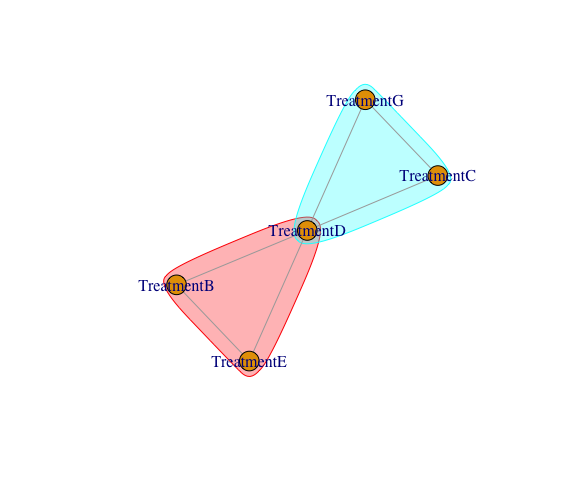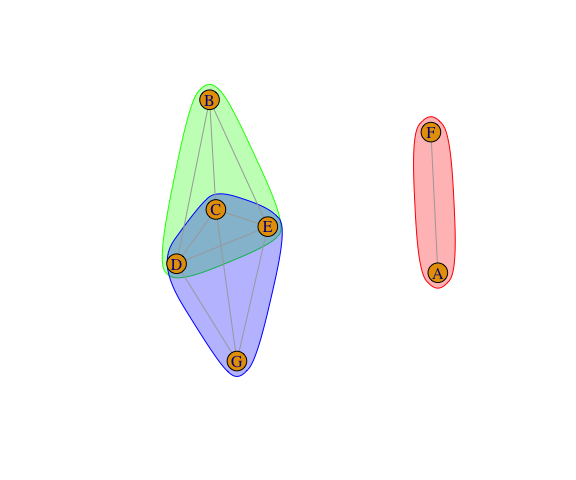# Post-hoc Analysis Using the Package postHoc

#### Spring 2020

library(postHoc)

We illustrate here some very basic post hoc analysis techniques implemented in the package postHoc.

#### A Simple Example

Consider the following data-frame supplied with postHoc.

str(DeIdentifiedExample)
#> 'data.frame':    70 obs. of  2 variables:
#>  $Y : num 9.83 7.39 10.22 11.83 9.03 ... #>$ Treatment: Factor w/ 7 levels "A","B","C","D",..: 1 1 1 1 1 1 1 1 1 1 ...

Here, Y is a response variable and Treatment is a classification variable with $$7$$ levels. There are $$10$$ replicates for each level of Treatment.

table(DeIdentifiedExample$Treatment) #> #> A B C D E F G #> 10 10 10 10 10 10 10 ###### A Gaussian one-way classification model We use a Gaussian one-way classification model (i.e., an ANOVA model) for describing these data and testing whether there is an effect of Treatment. Formally, we define the model refered above in the following way. Let $$Y_{t,r}$$ be a random variable representing the response of the $$r^\mbox{th}$$ replicate ($$r = 1, ..., 10$$) of the experimental units subjected to the $$t^\mbox{th}$$ treatment ($$t = A, ..., G$$). The model assumes that, $$Y_{A, 1}, ... , Y_{G, 10}$$ are independent and that $Y_{t,r} \sim N(\mu_t, \sigma^2), \mbox{ for } t = A, ..., G \mbox{ and } r = 1, ..., 10 \, .$ This model, termed the full model, is adjusted in the following way. FULLmodel <- lm(Y ~ Treatment + 0, data = DeIdentifiedExample) coef(FULLmodel) #> TreatmentA TreatmentB TreatmentC TreatmentD TreatmentE TreatmentF #> 10.154634 16.290514 13.546256 14.517146 16.183416 8.677142 #> TreatmentG #> 13.506536 Comparing the model above with a null model given by $Y_{t,r} \sim N(\mu, \sigma^2), \mbox{ for } t = A, ..., G \mbox{ and } r = 1, ..., 10 \, ,$ with the full model allow us to test the null hypothesis $H_0 : \mu_A = \mu_B = \mu_C = \mu_D = \mu_E = \mu_F = \mu_G$ See the execution below. NULLmodel <- lm(Y ~ 1, data = DeIdentifiedExample) anova(NULLmodel, FULLmodel) #> Analysis of Variance Table #> #> Model 1: Y ~ 1 #> Model 2: Y ~ Treatment + 0 #> Res.Df RSS Df Sum of Sq F Pr(>F) #> 1 69 757.91 #> 2 63 256.92 6 500.99 20.474 3.898e-13 *** #> --- #> Signif. codes: 0 '***' 0.001 '**' 0.01 '*' 0.05 '.' 0.1 ' ' 1 Since the p-value of the F-test below is very small we reject the null hypothesis $$H_0$$ and conclude that there is at least one pair $$(i,j)$$ (with $$i,j= A, ..., G$$) such that $$\mu_i \ne \mu_j$$. ###### Pairwise comparisons using the one-way classification model Once established that the means of the responses for the different treatments are not all equal, we might want to identify all the pairs that are different using techniques of post-hoc analysis. The funtion posthoc of the package postHoc calculates the p-values for the differences of each pair of levels of the factor Treatment and group the levels of Treatment in groups of significance (given a pre-fixed significance level, here taken as $$0.05$$). See the execution below. TT <- posthoc(Model = FULLmodel, EffectLabels = LETTERS[1:7], digits = 1) summary(TT) #> Est. 2.5% 97.5% Group A B C D E F #> A 10.2 8.9 11.4 a #> B 16.3 15.0 17.5 c 0 #> C 13.5 12.3 14.8 bc 0 0.1 #> D 14.5 13.3 15.8 bc 0 0.4 0.9 #> E 16.2 14.9 17.4 bc 0 1 0.1 0.5 #> F 8.7 7.4 9.9 a 0.7 0 0 0 0 #> G 13.5 12.3 14.8 b 0 0 1 0.9 0.1 0 The function posthoc uses a model as argument (here the “FULLmodel”) and returns an object (here “TT”) for which we can extract a range of information suitable for post hoc analysis. Here is a suite of those methods. print(TT) #> Levels ParameterCI #> 1 A 10.2(8.9-11.4)a #> 2 B 16.3(15-17.5)c #> 3 C 13.5(12.3-14.8)bc #> 4 D 14.5(13.3-15.8)bc #> 5 E 16.2(14.9-17.4)bc #> 6 F 8.7(7.4-9.9)a #> 7 G 13.5(12.3-14.8)b barplot(TT, ylim = c(5,21)) abline(h = 5)lines(TT, ylim = c(5,21))###### How posthoc constructs the grouping The function posthoc performs all the tests between pairs of the parameters of the models that are listed in the parameter EffectIndices. For example, the following calculations perform the tests for the tretments “B”, “C”,“D”, “E” and “G”. ZZ <- posthoc(Model = FULLmodel, EffectIndices = c(2,3,4,5,7), digits = 2) The object ZZ stores, among other things, the p-values of pairwise comperisons between those treatments. See below. ZZ$PvaluesMatrix
#>            TreatmentB TreatmentC TreatmentD TreatmentE TreatmentG
#> TreatmentB         NA         NA         NA         NA         NA
#> TreatmentC 0.02756079         NA         NA         NA         NA
#> TreatmentD 0.29560846 0.81860443         NA         NA         NA
#> TreatmentE 0.99995410 0.03773187  0.3575558         NA         NA
#> TreatmentG 0.02451703 0.99999913  0.7959406 0.03363749         NA

Having computed all the p-values of all the possible pairs of the selected treatments, an undirected graph is constructed (here graph reffers to the mathematical structure componsed of vertices, or points, and edges, or lines connecting the points), using the following conventions. The vertices represent the fixed effects in the model (here the treatments), and two vertices are connected by an edge when the corresponding parameters in the model are not statistically significanltnly different at the pre-fixed signicance (here we use the default of $$0.05$$). See below.

set.seed(143)
plot(ZZ)The idea explored for classifying the treatments is to calculate all the maximal cliques in the representation graph. Here a clique is a set of vertices (treatments) for wich all their elements are connected by an edge. A clique is maximal when it is not a subset of a larger clique. Examining the graph despected above it is easy to see that $$\{ B, D, E \}$$ and $$\{C, D, G \}$$ are both maximal cliques in the graph above. These cliques are identified in the figure above by drawing regions with different colours. The maximal cliques form the groups of significantly different treatments. See below.

summary(ZZ)
#>             Est.  2.5% 97.5% Group TreatmentB TreatmentC TreatmentD
#> TreatmentB 16.29 15.04 17.54     b
#> TreatmentC 13.55 12.29 14.80     a       0.03
#> TreatmentD 14.52 13.27 15.77    ab        0.3       0.82
#> TreatmentE 16.18 14.93 17.44     b          1       0.04       0.36
#> TreatmentG 13.51 12.25 14.76     a       0.02          1        0.8
#>            TreatmentE
#> TreatmentB
#> TreatmentC
#> TreatmentD
#> TreatmentE
#> TreatmentG       0.03

Note that the number of pairs to be compared in a post-hoc analysis growths very quickly with the number of effects studied (there are $$n(n-1)/2$$) possible pairs of $$n$$ objects); therefore, very complex patters can appear in post-hoc analyses, which might be difficult to see by nacked eyes. Fortunately, there are effective algoritmes to identify maximal cliques. See the example below constructed with all the $$7$$ treatments (yielding $$7 . 6/2 = 21$$ pairwise comparisons).

set.seed(1243)
plot(TT)